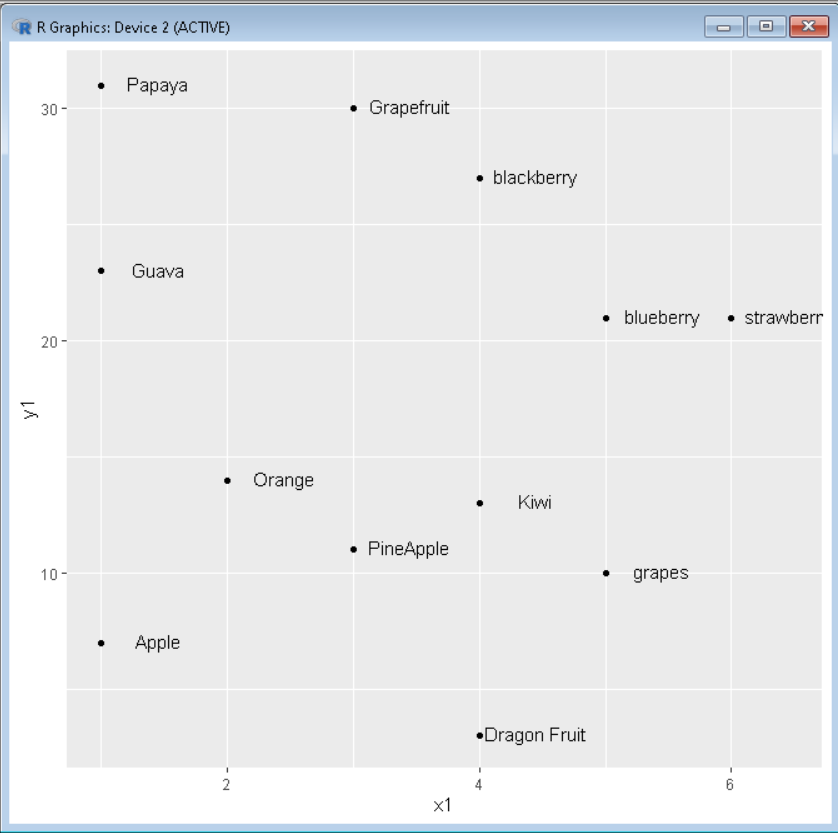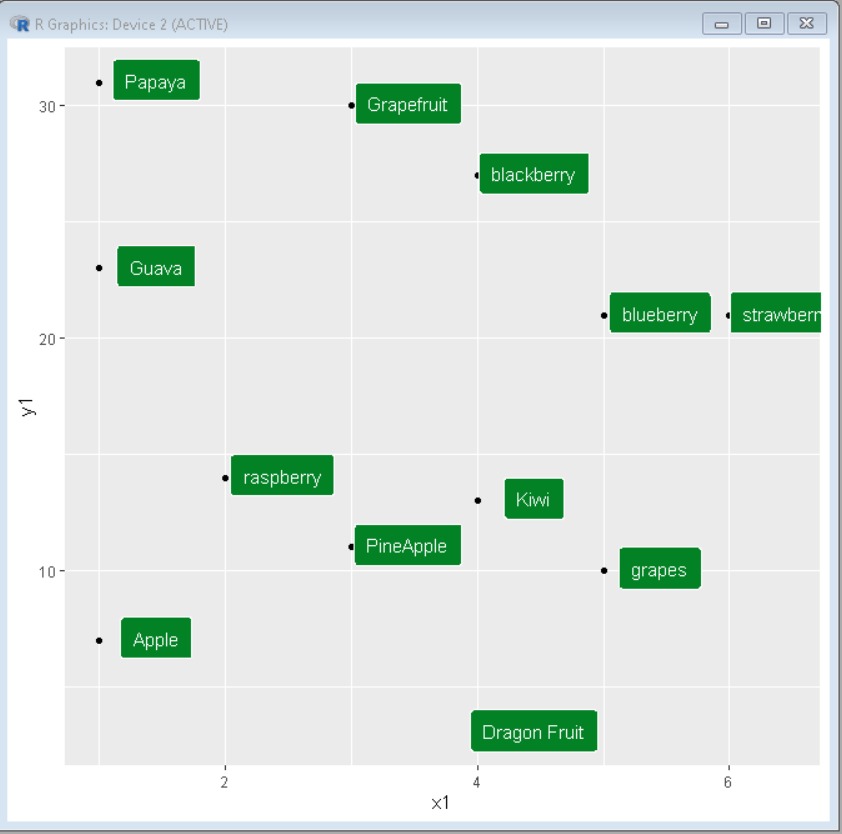Related Articles

# How to Add Labels Directly in ggplot2 in R

• Last Updated : 31 Aug, 2021

Labels are textual entities that have information about the data point they are attached to which helps in determining the context of those data points. In this article, we will discuss how to directly add labels to ggplot2 in R programming language.

To put labels directly in the ggplot2 plot we add data related to the label in the data frame. Then we use functions geom_text() or geom_label() to create label beside every data point. Both the functions work the same with the only difference being in appearance. The geom_label() is a bit more customizable than geom_text().

## Method 1: Using geom_text()

This method is used to add Text labels to data points in ggplot2 plots. It positions in the same manner as geom_point() does.

Syntax: ggp + geom_text( label,  nudge_x , nudge_y,   check_overlap )

Parameters:

• label: Text labels we want to show at data points
• nudge_x: shifts the text along X-axis
• nudge_y: shifts the text along Y-axis
• check_overlap:  avoids text overlap

Example: Scatter plot with labels on it using ggplot2 and geom_text.

## R

 `# import library ggplot2``library``(ggplot2)`` ` `# Create dataset``x1 < - ``c``(1, 1, 1, 2, 3, 4, 4, 4, 5, 5, 6, 2, 3)``y1 < - ``c``(7, 23, 31, 14, 11, 3, 13, 27, 21, 10, 21, 14, 30)``label1 < - ``c``(``'Apple'``, ``'Guava'``, ``'Papaya'``, ``'Orange'``, ``'PineApple'``,``             ``'Dragon Fruit'``, ``'Kiwi'``, ``'blackberry'``, ``'blueberry'``,``             ``'grapes'``, ``'strawberry'``, ``'raspberry'``, ``'Grapefruit'``)`` ` `sample_data < - ``data.frame``(x1, y1, label1)`` ` `# add text with geom_text``ggplot``(sample_data, ``aes``(x=x1, y=y1)) +``geom_point``() +``geom_text``(``    ``label=label1,``    ``nudge_x=0.45, nudge_y=0.1,``    ``check_overlap=T``)`

Output:## Method 2: Using geom_label()

This method is used to add Text labels to data points in ggplot2 plots. It pretty much works the same as the geom_text the only difference being it wraps the label inside a rectangle.

Syntax: ggp + geom_label( label,  nudge_x , nudge_y,   check_overlap,  label.padding,  label.size,  color,  fill )

Parameters:

• label: Text labels we want to show at data points
• nudge_x: shifts the text along X-axis
• nudge_y: shifts the text along Y-axis
• check_overlap:  avoids text overlap
• label.size: size of rectangular overlap
• color: color of text in label
• fill: background color of rectangular overlap

Example: Scatter plot with labels on it using ggplot2 and geom_label().

## R

 `# import library ggplot2``library``(ggplot2)`` ` `# Create dataset``x1 < - ``c``(1, 1, 1, 2, 3, 4, 4, 4, 5, 5, 6, 2, 3)``y1 < - ``c``(7, 23, 31, 14, 11, 3, 13, 27, 21, 10, 21, 14, 30)``label1 < - ``c``(``'Apple'``, ``'Guava'``, ``'Papaya'``, ``'Orange'``, ``'PineApple'``,``             ``'Dragon Fruit'``, ``'Kiwi'``, ``'blackberry'``, ``'blueberry'``,``             ``'grapes'``, ``'strawberry'``, ``'raspberry'``, ``'Grapefruit'``)`` ` `sample_data < - ``data.frame``(x1, y1, label1)`` ` `# add text with geom_text``ggplot``(sample_data, ``aes``(x=x1, y=y1)) +``geom_point``() +``geom_label``(``    ``label=label1,``    ``nudge_x=0.45, nudge_y=0.1,``    ``check_overlap=T,``    ``label.padding=``unit``(0.55, ``"lines"``),``    ``label.size=0.4,``    ``color=``"white"``,``    ``fill=``"#038225"``)`

Output:My Personal Notes arrow_drop_up